1560864309

# Introducing PyTorch BigGraph

Facebook’s New Framework for Processing Large Graphs. Graphs are one of the fundamental data structures in machine learning applications.

Specifically, graph-embedding methods are a form of unsupervised learning, in that they learn representations of nodes using the native graph structure. Training data in mainstream scenarios such as social media predictions, internet of things(IOT) pattern detection or drug-sequence modeling are naturally represented using graph structures. Any one of those scenarios can easily produce graphs with billions of interconnected nodes. While the richness and intrinsic navigation capabilities of graph structures is a great playground for machine learning models, their complexity posses massive scalability challenges. Not surprisingly, the support for large-scale graph data structures in modern deep learning frameworks is still quite limited. Recently, Facebook unveiled PyTorch BigGraph, a new framework that makes it much faster and easier to produce graph embeddings for extremely large graphs in PyTorch models.

To some extent, graph structures can be seen as an alternative to labeled training dataset as the connections between the nodes can be used to infer specific relationships. This is the approach followed by unsupervised graph embedding methods which learn a vector representation of each node in a graph by optimizing the objective that the embeddings for pairs of nodes with edges between them are closer together than pairs of nodes without a shared edge. This is similar to how word embeddings like word2vec are trained on text.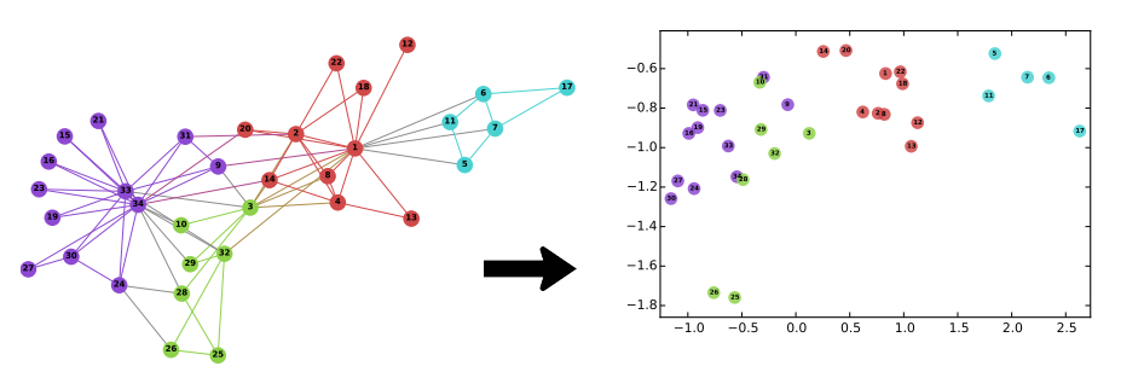Most graph embedding methods result quite constrained when applied to large graph structures. To give a example, a model with two billion nodes and 100 embedding parameters per node (expressed as floats) would require 800GB of memory just to store its parameters, thus many standard methods exceed the memory capacity of typical commodity servers. To represents a major challenge for deep learning models and is the genesis of Facebook’s BigGraph framework.

### PyTorch BigGraph

The goal of PyTorch BigGraph(PBG) is to enable graph embedding models to scale to graphs with billions of nodes and trillions of edges. PBG achieves that by enabling four fundamental building blocks:

• graph partitioning, so that the model does not have to be fully loaded into memory
• multi-threaded computation on each machine
• distributed execution across multiple machines (optional), all simultaneously operating on disjoint parts of the graph
• batched negative sampling, allowing for processing >1 million edges/sec/machine with 100 negatives per edge

PBG addresses some of the shortcomings of traditional graph embedding methods by partitioning the graph structure into randomly divided into P partitions that are sized so that two partitions can fit in memory. For example, if an edge has a source in partition p1 and destination in partition p2 then it is placed into bucket (p1, p2). In the same model, the graph edges are then divided into P2 buckets based on their source and destination node. Once the nodes and edges are partitioned, training can be performed on one bucket at a time. The training of bucket (p1, p2) only requires the embeddings for partitions p1 and p2 to be stored in memory. The PBG structure guarantees that buckets have at least one previously-trained embedding partition.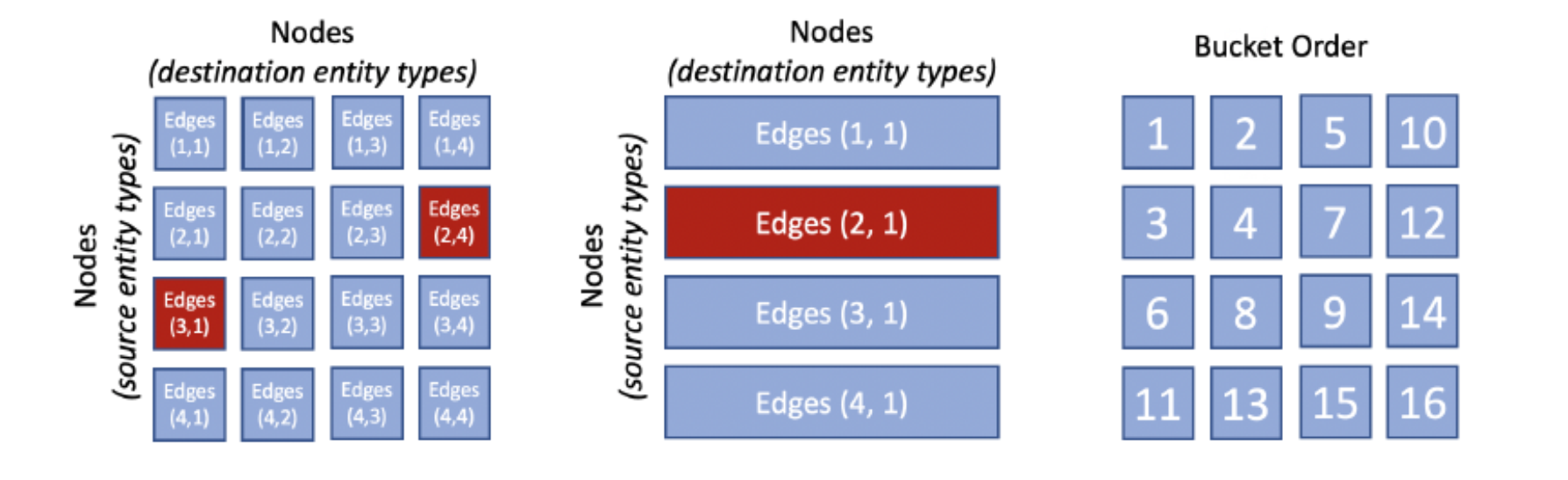Another area in which PBG really innovates is the parallelization and distribution of the training mechanics. PBG uses PyTorch parallelization primitives to implement a distributed training model that leverages the block partition structure illustrated previously. In this model, individual machines coordinate to train on disjoint buckets using a lock server which parcels out buckets to the workers in order to minimize communication between the different machines. Each machine can train the model in parallel using different buckets.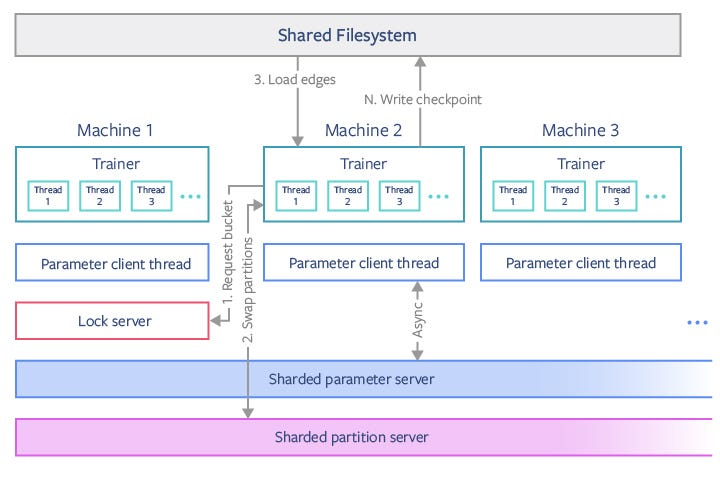In the previous figure, the Trainer module in machine 2 requests a bucket from the lock server on machine 1, which locks that bucket’s partitions. The trainer then saves any partitions that it is no longer using and loads new partitions that it needs to and from the sharded partition servers, at which point it can release its old partitions on the lock server. Edges are then loaded from a shared filesystem, and training occurs on multiple threads without inter-thread synchronization. In a separate thread, a small number of shared parameters are continuously synchronized with a sharded parameter server. Model checkpoints are occasionally written to the shared filesystem from the trainers. This model allows a set of P buckets to be parallelized using up to P/2 machines.

One of the indirect innovations of PBG is the use of batched negative sampling techniques. Traditional graph embedding models, construct random “false” edges as negative training examples along with the true positive edges. This significantly speeds training because only a small percentage of weights must be updated with each new sample. However, the negative samples end up introducing a performance overhead in the processing of the graph and end up “corrupting” true edges with random source or destination nodes. PBG introduces a method that reuses a single batch of N random nodes to produce corrupted negative samples for N training edges. In comparison to other embedding methods, this technique allows us to train on many negative examples per true edge at little computational cost.

To increase memory efficiency and computational resources on large graphs, PBG leverages a single batch of Bn sampled source or destination nodes to construct multiple negative examples.In a typical setup, PBG takes a batch of B = 1000 positive edges from the training set, and breaks it into chunks of 50 edges. The destination (equivalently, source) embeddings from each chunk is concatenated with 50 embeddings sampled uniformly from the tail entity type. The outer product of the 50 positives with the 200 sampled nodes equates to 9900 negative examples.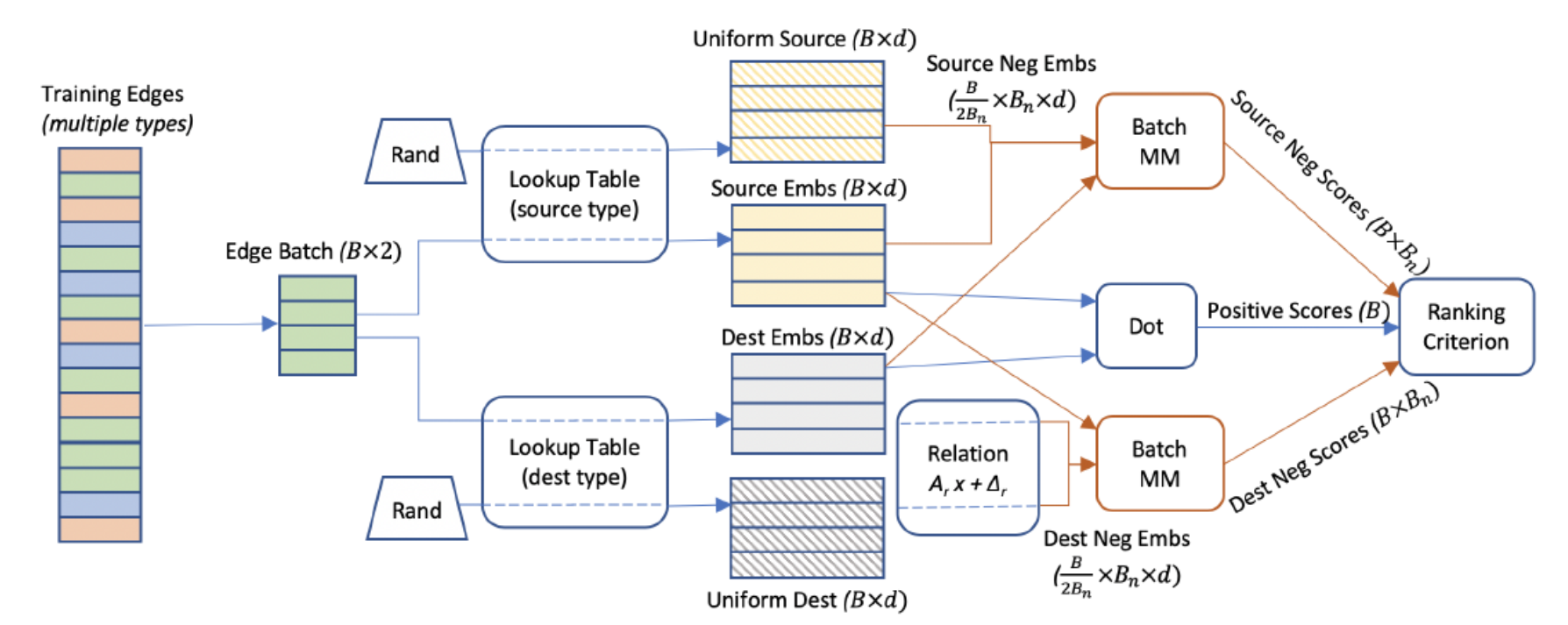The batched negative sampling approach has a direct impact in the speed of the training of the models. Without batching, the speed of training is inversely proportional to the number of negative samples. Batched training improves that equation achieving constant training speed.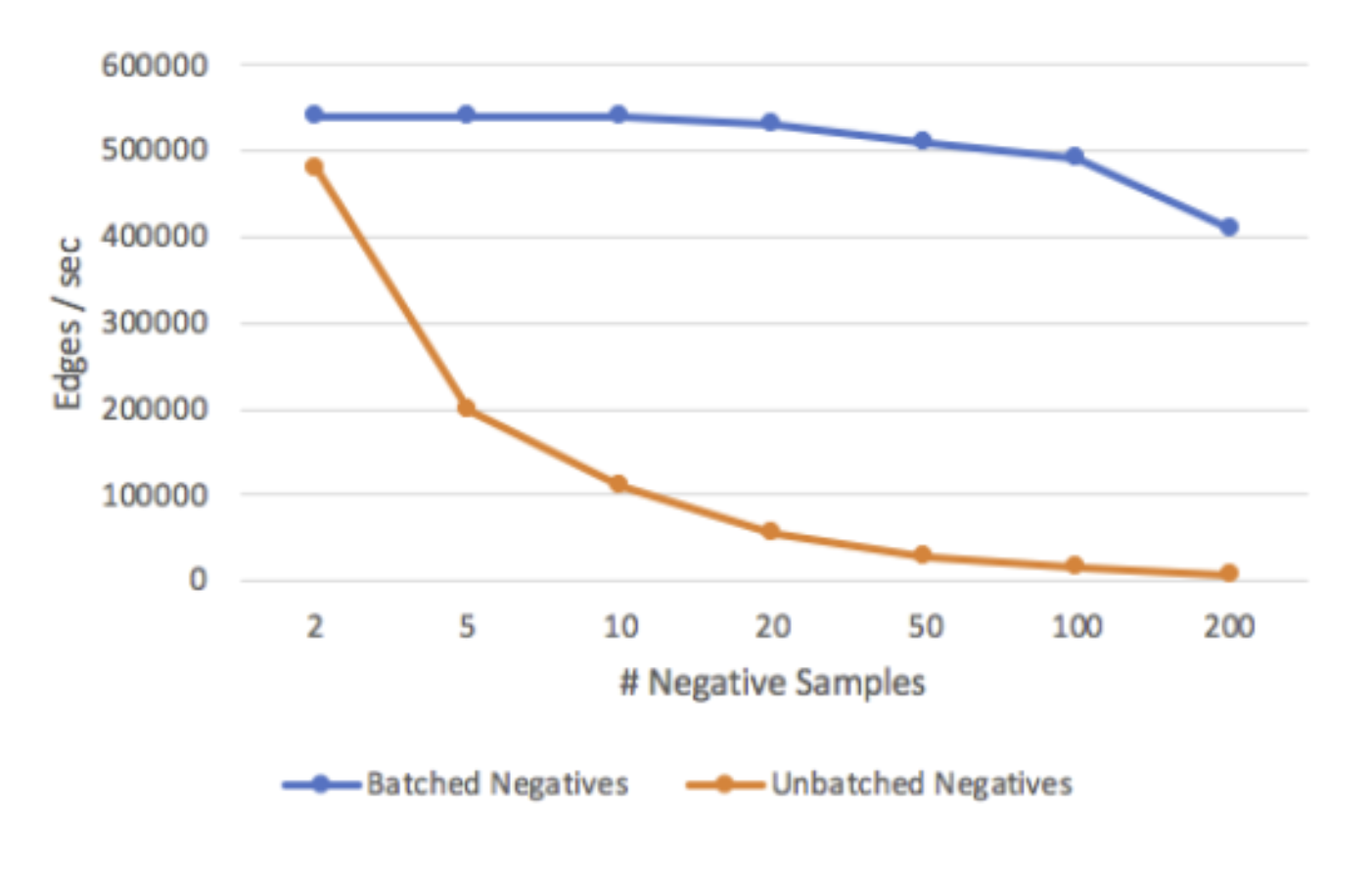Facebook evaluated PGB using different graph datasets such as LiveJournal, Twitter data and YouTube user interaction data. Additionally, PBG was benchmarked using the Freebase knowledge graph, which contains more than 120 million nodes and 2.7 billion edges as well as a smaller subset of the Freebase graph, known as FB15k, which contains 15,000 nodes and 600,000 edges and is commonly used as a benchmark for multi-relation embedding methods. The FB15k experiments showed PBG performing similarly to state of the art graph embedding models. However, when evaluated against the full Freebase dataset, PBG show memory consumptions improves by over 88%.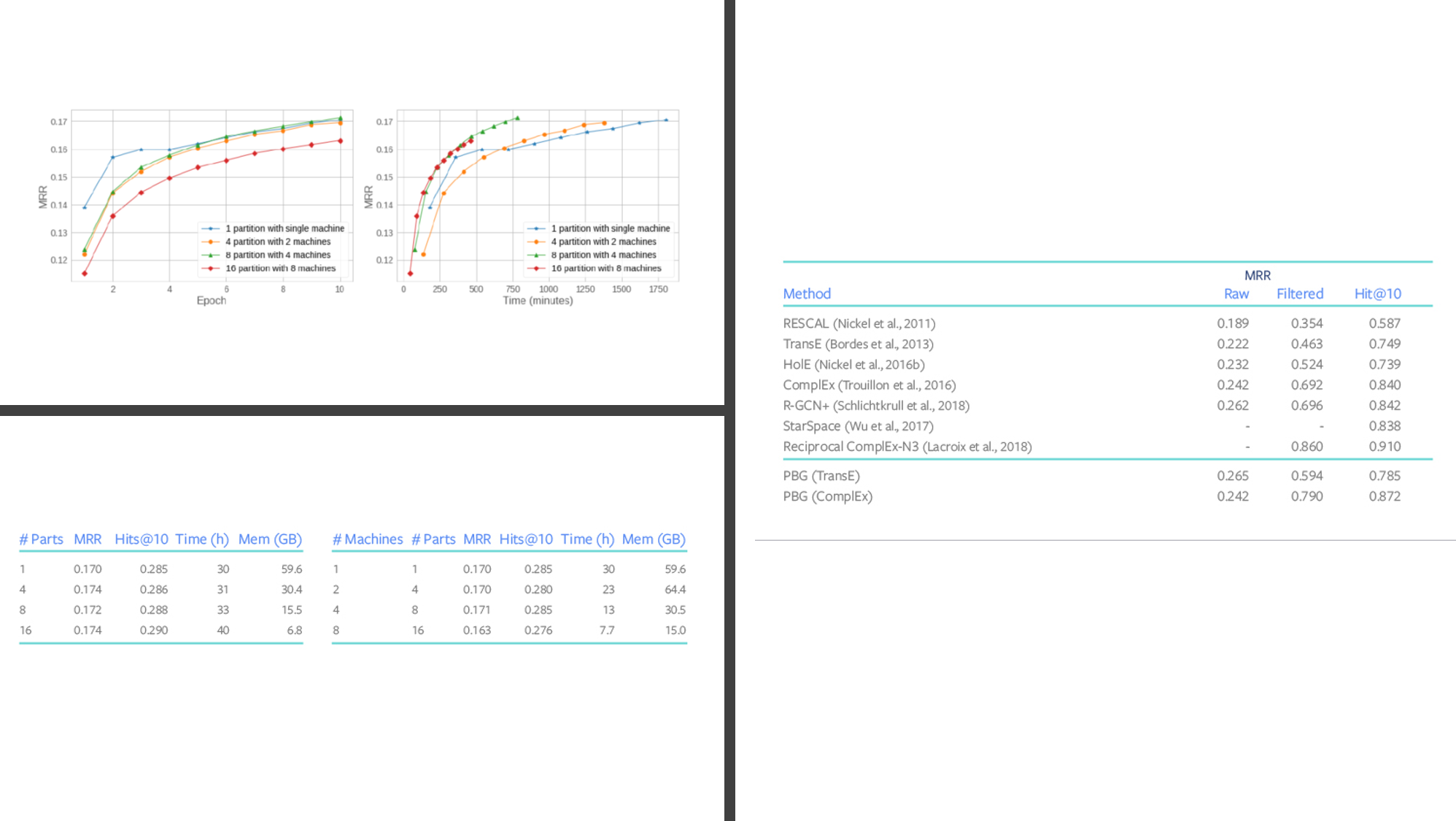PBG is one of the first methods that can scale and the training and processing of graph data to structures with billions of nodes and trillions of edges. The first implementation of PBG has been open sourced in GitHub and we should expect interesting contributions in the near future.

## Buddha Community1600632000

## What is Pytorch ?

Pytorch is a Deep Learning Library Devoloped by Facebook. it can be used for various purposes such as Natural Language Processing , Computer Vision, etc

## Prerequisites

Python, Numpy, Pandas and Matplotlib

## Tensor Basics

What is a tensor ?

A Tensor is a n-dimensional array of elements. In pytorch, everything is a defined as a tensor.

#pytorch #pytorch-tutorial #pytorch-course #deep-learning-course #deep-learning1599126480

## Facebook Gives Away This PyTorch Library For Differential Privacy

Recently, Facebook AI open-sourced a new high-speed library for training PyTorch models with differential privacy (DP) known as Opacus. The library is claimed to be more scalable than existing state-of-the-art methods.

According to the developers at the social media giant, differential privacy is a mathematically rigorous framework for quantifying the anonymisation of sensitive data. With the growing interest in the machine learning (ML) community, this framework is often used in analytics and computations.

Differential privacy constitutes a strong standard for privacy guarantees for algorithms on aggregate databases. It is usually defined in terms of the application-specific concept of adjacent databases. The framework has several properties that make it particularly useful in applications, such as group privacy, robustness to auxiliary information, among others.

#developers corner #differential privacy #facebook ai research #facebook differential privacy #opacus #pytorch #pytorch library #pytorch library opacus1610436416

## Guide to Conda for TensorFlow and PyTorch

Learn how to set up anaconda environments for different versions of CUDA, TensorFlow, and PyTorch

It’s a real shame that the first experience that most people have with deep learning is having to spend days trying to figure out why the model they downloaded off of GitHub just… won’t… run….

Dependency issues are incredibly common when trying to run an off-the-shelf model. The most problematic of which is needing to have the correct version of CUDA for TensorFlow. TensorFlow has been prominent for a number of years meaning that even new models that are released could use an old version of TensorFlow. This wouldn’t be an issue except that it feels like every version of TensorFlow needs a specific version of CUDA where anything else is incompatible. Sadly, installing multiple versions of CUDA on the same machine can be a real pain!

#machine-learning #pytorch #tensorflow #pytorch1603675380

## How to tune Pytorch Lightning hyperparameters

Pytorch Lightning is one of the hottest AI libraries of 2020, and it makes AI research scalable and fast to iterate on. But if you use Pytorch Lightning, you’ll need to do hyperparameter tuning.

Proper hyperparameter tuning can make the difference between a good training run and a failing one. Everyone knows that you can dramatically boost the accuracy of your model with good tuning methods!

In this blog post, we’ll demonstrate how to use Ray Tune, an industry standard for hyperparameter tuning, with PyTorch Lightning. Ray Tune is part of Ray, a library for scaling Python.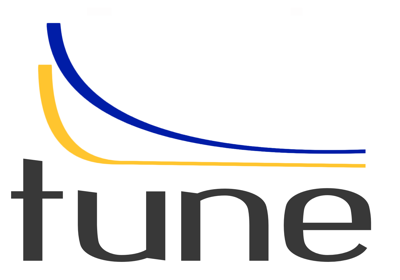It is available as a PyPI package and can be installed like this:

``````pip install "ray[tune]"
``````

To use Ray Tune with PyTorch Lightning, we only need to add a few lines of code!!

## Getting started with Ray Tune + PTL!

To run the code in this blog post, be sure to first run:

``````pip install "ray[tune]"
pip install "pytorch-lightning>=1.0"
pip install "pytorch-lightning-bolts>=0.2.5"
``````

_The below example is tested on ray==1.0.1 , pytorch-lightning==1.0.2, and pytorch-lightning-bolts==0.2.5. _See the full example here.

``````import torch
from torch.nn import functional as F
import pytorch_lightning as pl
from pl_bolts.datamodules import MNISTDataModule
import os
from ray.tune.integration.pytorch_lightning import TuneReportCallback
``````

After imports, there are three easy steps.

1. Create your `LightningModule`
2. Create a function that calls `Trainer.fit` with the Tune callback
3. Use `tune.run` to execute your hyperparameter search.

#hyperparameter-tuning #pytorch-lightning #data-science #pytorch1600642800

## PyTorch For Deep Learning — Confusion Matrix

Note: This is a regular classification problem with PyTorch and this is exactly like the one in the previous post of the “PyTorch for Deep Learning” series.

The Reason for doing writing the post is for some more reference to classification problem and better understanding. If You are already good enough with classification withneural network, skip to the part where confusion matrix comes in.

## Jumping to the Code Part

1. Importing required libraries
``````#importing the libraries

import torch
import numpy as np
import pandas as pd
import matplotlib.pyplot as plt
import seaborn as sns
``````

2. Data

The dataset is available at kaggle : https://www.kaggle.com/dragonheir/logistic-regression

``````#importing the dataset